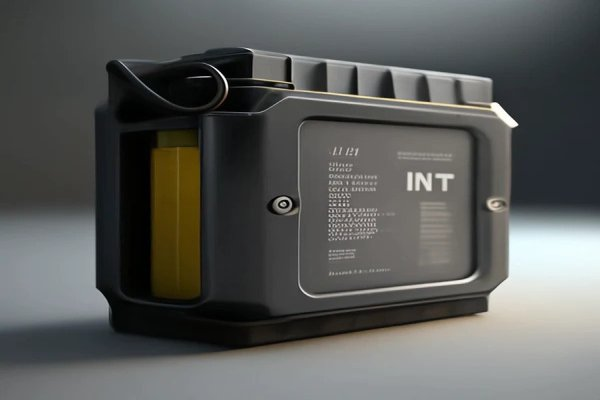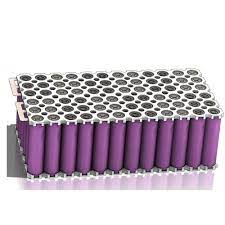# How to calculate how many battery cells in battery?

Front Fork with Suspension VS Solid:Which Is Right for Me? Reading How to calculate how many battery cells in battery? 3 minutes Next Ebike Markets: Europe vs. the USA

To know how electric bike factory calculate how many battery customer need, first we need to have the basic knowledge of Series Circuit and Parallel circuit.Series Circuit

In a series circuit, the current remains constant because there is only one path for it to flow.

When current passes through a resistor in a series circuit, a voltage drop is produced, and therefore, the voltage is distributed among the resistors.

The total voltage is equal to the sum of the voltages across each resistor. V_total = V1+V2+…+Vn.

Parallel circuit

The total current is equal to the sum of the currents in each parallel branch. I_total = I1+I2+...+In.

In a parallel circuit, the capacitance adds up to the total capacitance. C_total = C1+C2+...+Cn.

In parallel circuits, the voltage remains constant, and each branch has the same voltage.

If we have a parallel circuit with two elements having voltages V1 and V2, the total voltage would be V1 = V2.

From the above, we know that for series circuit, the current does not change, but V_total = V1+V2+…+Vn.. For parallel circuit, Voltage does not change, but I_total = I1+I2+...+In. Also the capacitance adds up to the total capacitance. C_total = C1+C2+...+Cn.

Battery cell

Also we need to know all the battery are made of small battery cell which usually consists of positive and negative electrodes, a separator, andan electrolyte. The two common type battery cells are 18650 battery cell and 21700 battery cell with voltage of 3.6V or 3.7V. 18650’s capacitance is usually 2200mAh to 3500mAh, while 21700’s capacitance is 5000mAh. It makes 21700 battery cell is more expensive than 18650’s one.

With all above knowledge, I will teach you have to calculate the battery’s capacitance with a known number of battery cells and voltage.

Quesiton 1:

The customer wants 70 18650 battery cells, with 36V of total voltage, what is the battery’s total capacitance?

With the known 18650 battery cells, we set the voltage of each is 3.6V, and capacitance is 3500mAh.

36V/3.6V = 10 series circuit

We have 70 battery cells, 70/10 = 7 parallel circuit

The capacitance only add to total in parallel circuit, we have 7* 3500mAh = 24,500 mAh

Question 2

The customer wants a total current of 25A and a total voltage of 52V, how many 18650 battery cells are needed?

With the known 18650 battery cells, we set the voltage of each is 3.6V, and capacitance is 3500mAh.

52V/3.6V = 14 series circuit

Our total current = 25A, 25A/14 = 2

2*14 =28

We need 28 18650battery cells in conclusion.